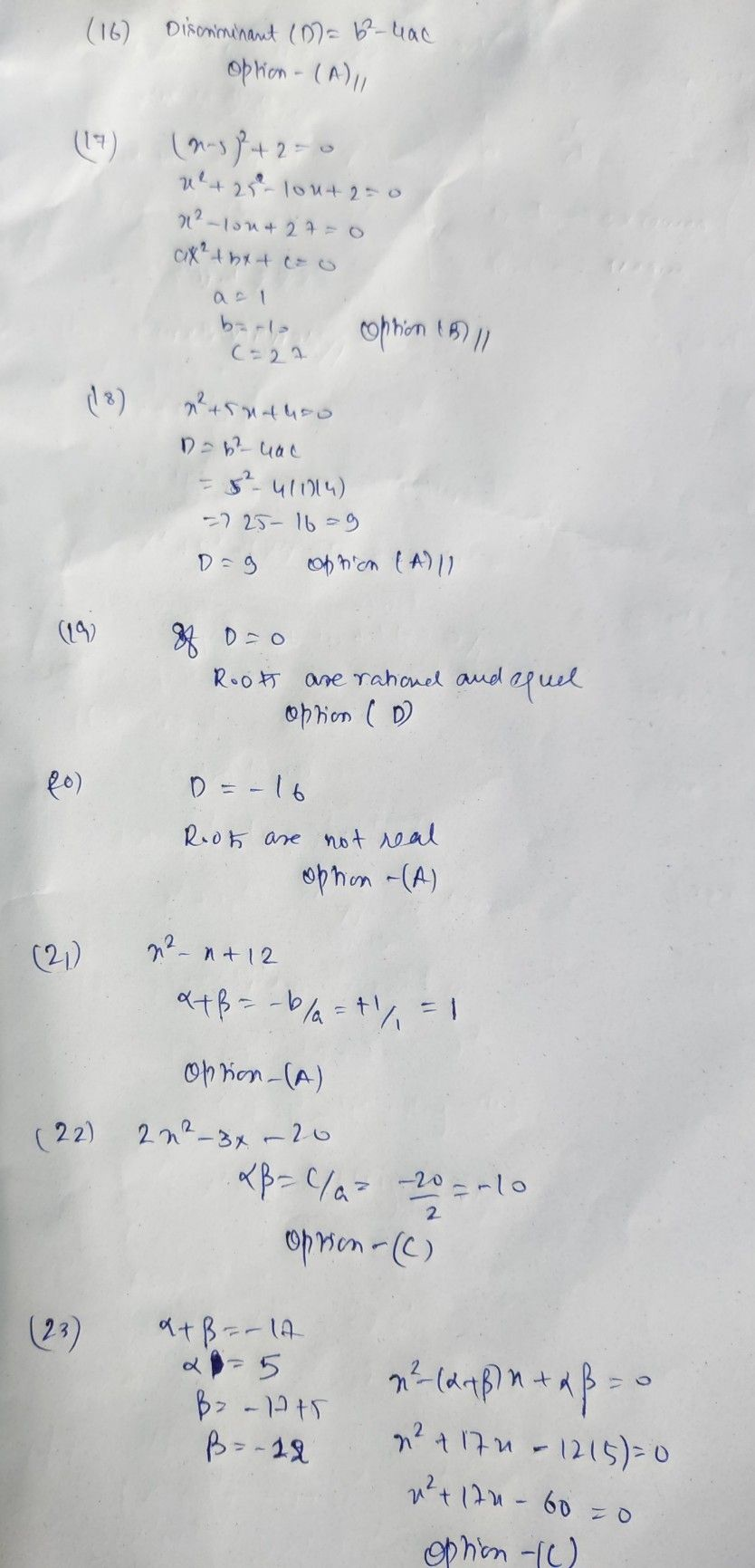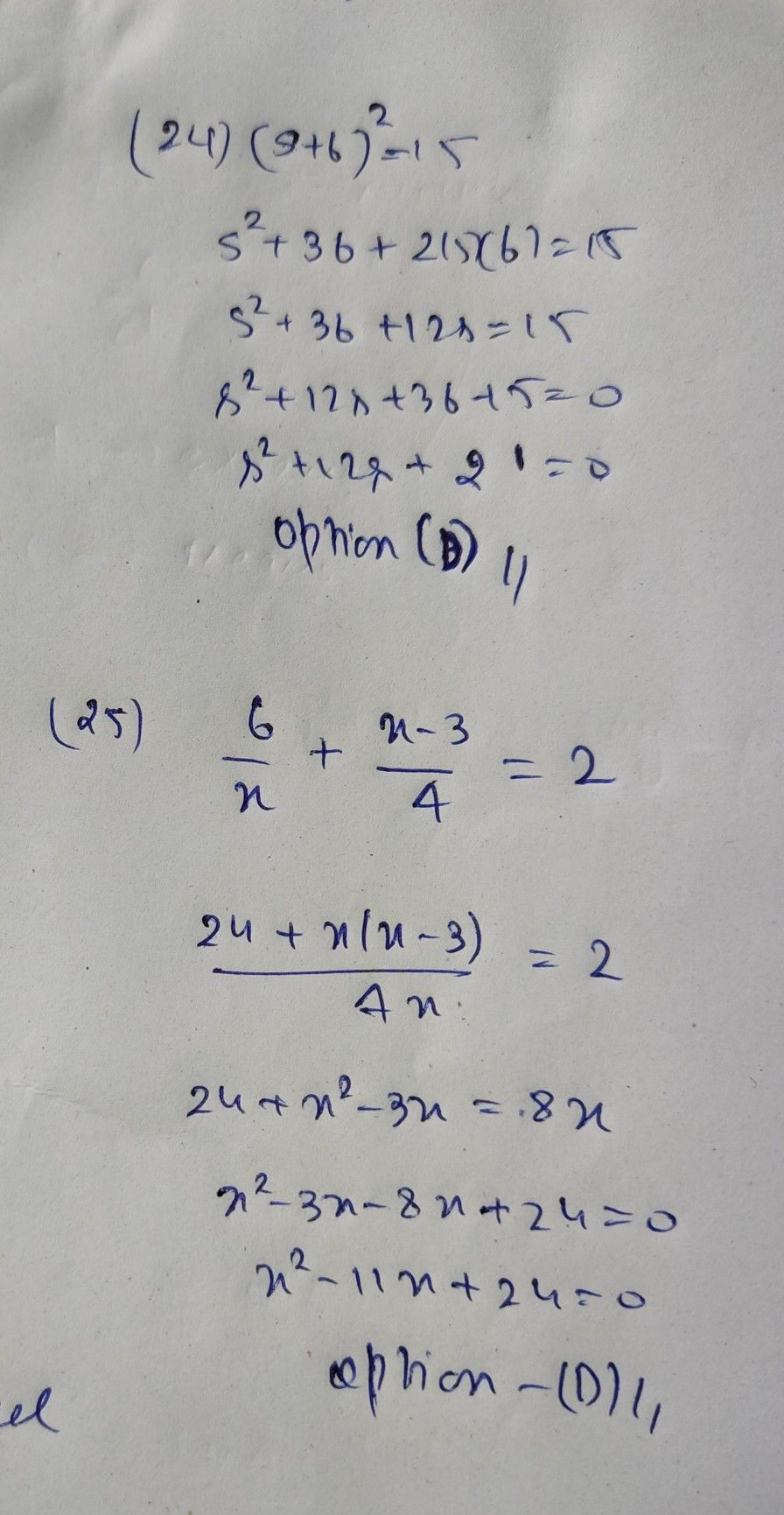Symbol
Problem16. Which of $a009$ $a$ 16. ABCD equation? the following expressijons is used to Bnd the discriminant of a quat ae A. b2-4ac B. b2 + 4ac $b=4$ 17. The value of a, b. and cin the quadratic equation $0$ $b+4ac$ A. 1, 10, 27 (x-5) $2=0xcspccta$ $a1y$ $90°0$ $0c$ $0$ $o00$ $8c$ $0$ B. 1.-10, 27 D. C. 1, 10, -27 18. mat $1$ $-10$ $.27$ A is the value of the discriminant of the quadratic equation x + 5x +4=07 9 C. 41 B. -9 D. -41 $00019$ $n$ $c$ $0$ mt is the nature of the roots of the quadratic equation if the value of its discriminant is zero? A. The roots are not real. C. The roots are irrational and not equal. B. The roots are rational and not equal. D. The roots are rational OOOO 20. What is the nature of the roots of a quadratic equation if the discriminant is -167 and equal. ABCD BA . The roots are not real. C. The roots are irrational and not equal. The roots are rational and not equal. D. The roots are rational and equal. $\square 0O21$ What is the sum of the roots of the quadratic equation x2 - x - 12 = 07 ABCD 1 C. -12 BA. . -1 D. 12 OOOO22. What is the product of the roots of the quadratic equation $2x2-3x-20=07$ ABCD C. -10 A. - 3 3 D. 10 B. $OOOO23$ $A$ $Bc$ $D$ The sum of the roots of a quadratic equation is -17. If one of the roots is 5, what could be the equation? x2 - 17x - 60 = 0 AB. . $x^{2}+17x+60=0$ CD. . $x^{2}+17x-60=0$ x2 - $\dfrac {17x+60=0} {0\left(s+6\right)^{2}=15}$ O24. Which of the following quadratic equation is equivalent $OOOO$ $A$ $BcD$ s2 - 12s - 21 = 0 C. s2 - $12s+21$ = 0 BA. . s2 + 12s - 21 = 0 D. $s^{2}+12s+21$ = 0 O 25. Which of the following quadratic equations is equivalent with the rational algebraic equatior $ABC$ D °+ X-3 = 2? 4 A. x2 - 11x - 24 = 0 C. $x^{2+11x+24}=0$ $x^{2}-11x+24=0$ B. x2 + 11x - 24 = 0 D.